: The Digits Cube Sum : Curious Properties of Reiterated : Curious Properties of Reiterated

# 1. The Collatz Problem

One of the most well known reiterated process is the Collatz Problem. See .
We are going to define Collatz function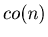for an arbitrary positive integer.

Definition 1.1.   We define Collatz fucntion by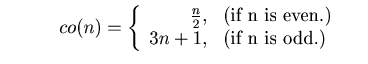Example 1.1.   By using the Mathematica function in Example 10.1 we can study this problem. For example, if we apply Collatz function co(n) to the number 156 repeatedly by using Mathematica programs in Example 10.1 and 10.2, then we have

{156, 78, 39, 118, 59, 178, 89, 268, 134, 67, 202, 101, 304, 152, 76, 38, 19, 58, 29, 88, 44, 22, 11, 34, 17, 52, 26, 13, 40, 20, 10, 5, 16, 8, 4, 2, 1}. By Mathematica we can make a graph of the above sequence.

Graph 1.1.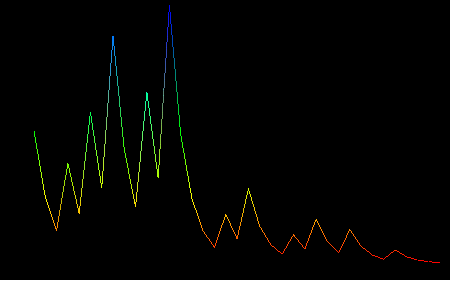Collatz Problem is to prove that the sequence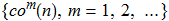eventually returns to 1 for any natural number n. This problem is a well known unsolved problem.
Example 1.2.   Even a relatively small number can have a long steps before arriving at 1. For example the number 27 needs 111 times to arrive at 1. See Graph 1.2.

Graph 1.2.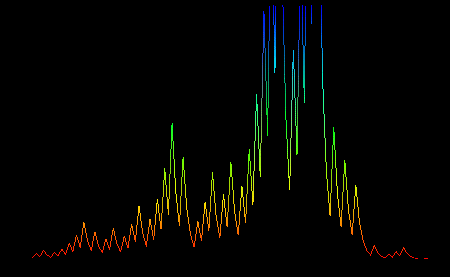According to  Collatz Problem has been tested and found to always reach 1 for all numbers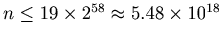.

Example 1.3.   According to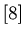the number less than 100 million with the longest total stopping time is 63728127 with 949 steps, and the number less than 1 billion with the longest total stopping time is 670617279 with 986 steps. By using the Mathematica function in Example 10.1 and Example 10.2 we can make the graphs of the sequences that start with 63728127 and 670617279 respectively. See Graph 1.3 and 1.4. The similarity between these graphs is remarkable.

Graph 1.3.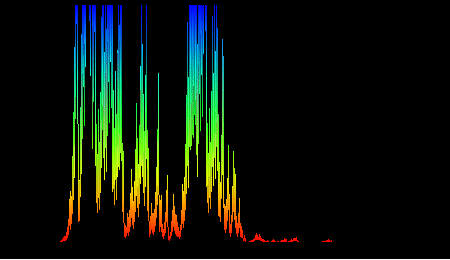Graph 1.4.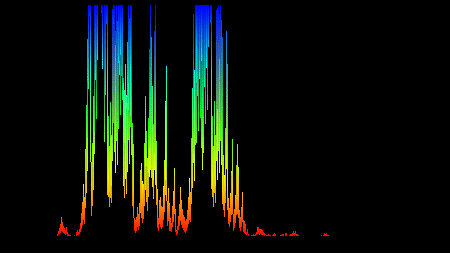: The Digits Cube Sum : Curious Properties of Reiterated : Curious Properties of Reiterated